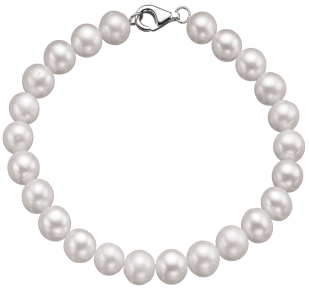# Pearl Bracelet

If you have a pearl bracelet, what is the ratio of the radius of the pearls to the radius of the bracelet? Clearly it depends on the number of pearls in the bracelet, but what is the relationship?We’ll make some assumptions:
• The pearls are perfectly spherical.
• The pearls touch each other with no gaps.
• There is no clasp/hasp.
• The band holding the pearls goes through the center of each pearl.
If you think about it, the center of each pearl forms is the vertex of a polygon on order n. We can draw a radius of the bracelet R to the center of each pearl, and make a right-triangle using the radius of the pearl P as the hypotenuse. The sine of the angle β is simply the ratio of the P/R.We also know that there are 2n lots of β around the circle, which has radians, so β = 2π/2n. Combining these, sin(π/n) = P/R, and this gives us our answer.